# Lesson 6 Linear Equations Solving MultiStep Equations WarmUp

• Slides: 11Lesson 6 Linear Equations Solving Multi-Step Equations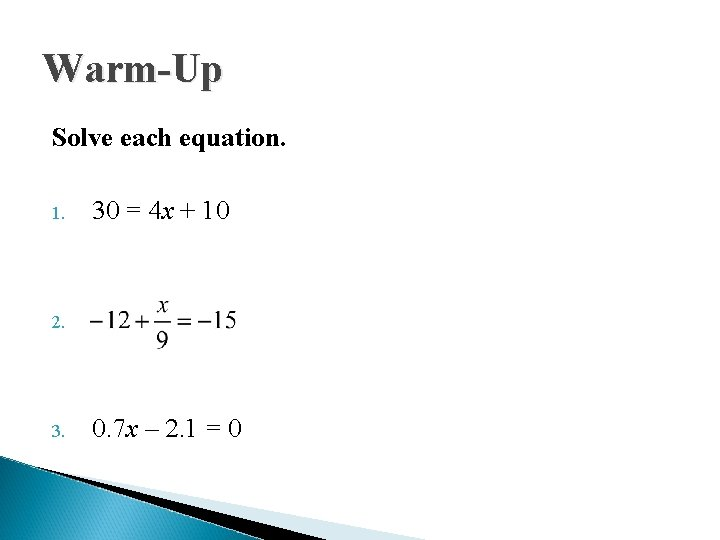Warm-Up Solve each equation. 1. 30 = 4 x + 10 2. 3. 0. 7 x – 2. 1 = 0Solving Multi-Step Equations Target: Simplify and solve multi-step equations.Solving Multi-Step Equations 1. Simplify each side of the equation by distributing and combining like terms, when necessary. 2. If variables are on both sides of the equation, balance the equation by moving one variable term to the opposite side of the equals sign using inverse operations. 3. Follow the process for solving one- and two-step equations to get the variable by itself.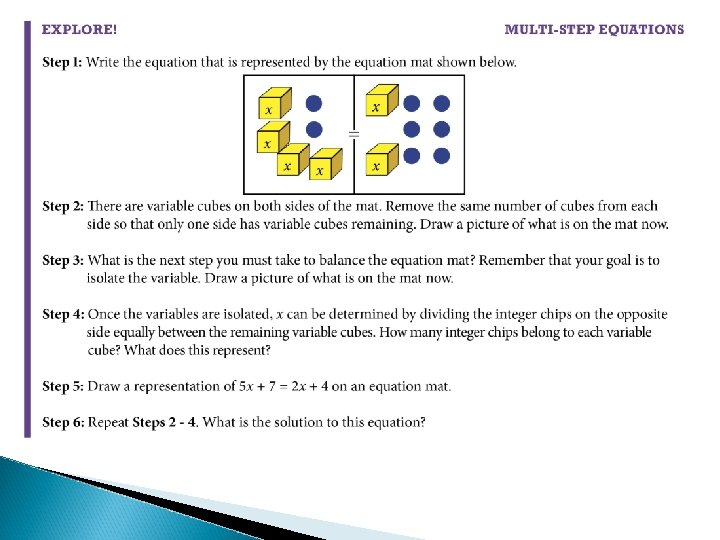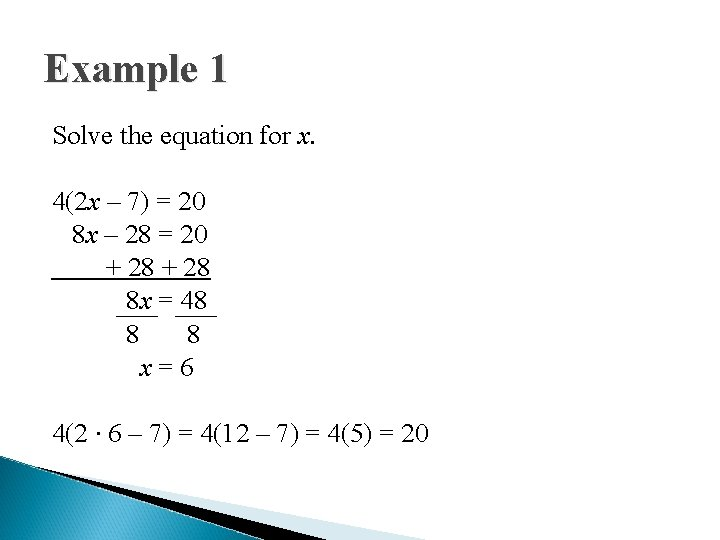Example 1 Solve the equation for x. 4(2 x – 7) = 20 8 x – 28 = 20 + 28 8 x = 48 8 8 x=6 4(2 ∙ 6 – 7) = 4(12 – 7) = 4(5) = 20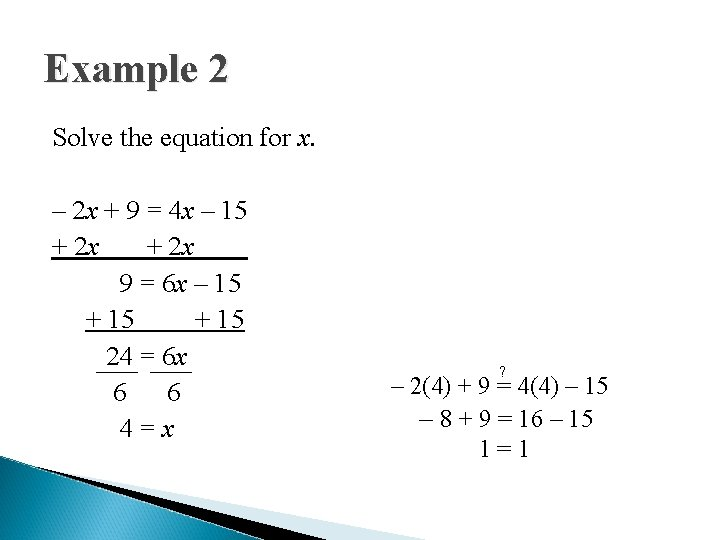Example 2 Solve the equation for x. – 2 x + 9 = 4 x – 15 + 2 x 9 = 6 x – 15 + 15 24 = 6 x 6 6 4=x ? – 2(4) + 9 = 4(4) – 15 – 8 + 9 = 16 – 15 1=1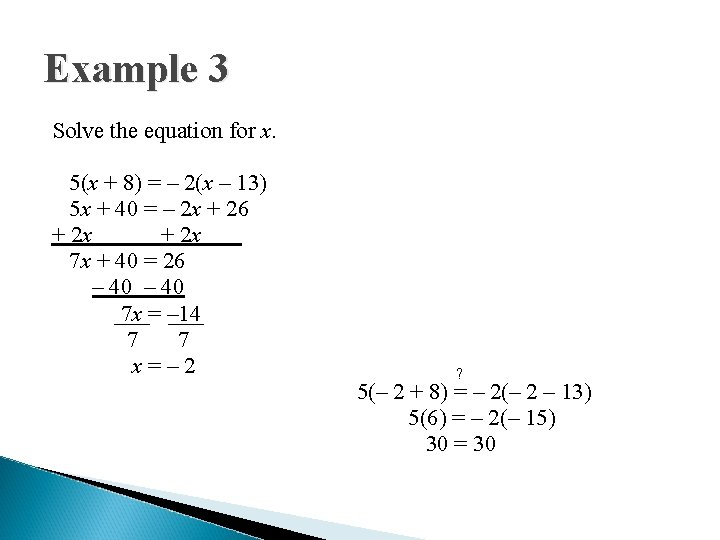Example 3 Solve the equation for x. 5(x + 8) = – 2(x – 13) 5 x + 40 = – 2 x + 26 + 2 x 7 x + 40 = 26 – 40 7 x = – 14 7 7 x=– 2 ? 5(– 2 + 8) = – 2(– 2 – 13) 5(6) = – 2(– 15) 30 = 30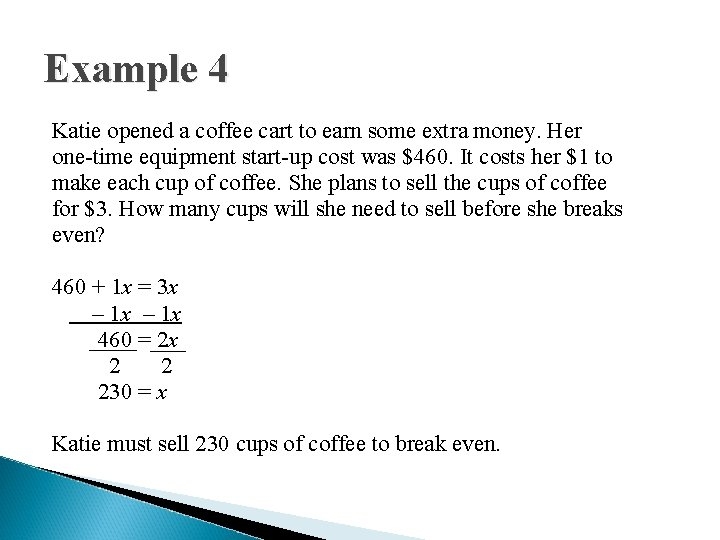Example 4 Katie opened a coffee cart to earn some extra money. Her one-time equipment start-up cost was \$460. It costs her \$1 to make each cup of coffee. She plans to sell the cups of coffee for \$3. How many cups will she need to sell before she breaks even? 460 + 1 x = 3 x – 1 x 460 = 2 x 2 2 230 = x Katie must sell 230 cups of coffee to break even.Exit Problems Solve each equation. 1. 90 = 10(x + 7) 2. 6 x − 13 = 4 x + 11 3. 2(x − 9) = 3(x − 5)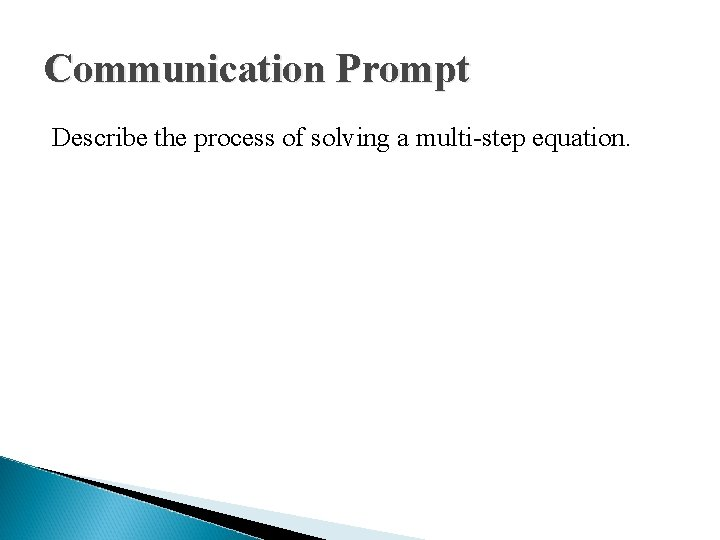Communication Prompt Describe the process of solving a multi-step equation.